/

### Function Description

Returns a plot of the asset mixes underlying a (constrained quadratic) efficient frontier. More precisely, returns a string which is the (temporary) code for a SmartChart that plots such information.

These asset mixes are the portfolios that optimise the following utility (for a variety of risk-reward trade-off factors,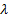), where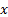is a vector of portfolio weights,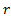is a vector of assumed returns on each asset and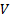is the covariance matrix (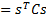, whereis the vector of risks on each asset class, here assumed to be characterised by their volatilities, as this approach is merely a mean-variance one, and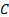their correlation matrix):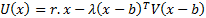Inputs are as per MnConstrainedQuadraticPortfolioOptimiser (which is the web function used to calculate the underlying portfolios contributing to the efficient frontier) except that:

(a)    An array of’s is supplied rather than a single value

(a)    The user can select a Title for the chart (using ChartTitle)

(b)   An additional ChartFormat parameter is available to specify how the chart should be formatted. For further details on how to use the ChartFormat parameter to format a chart see using the ChartFormat parameter.

Contents | Prev | Next